MORE IN Structural Analysis 1
VTU Civil Engineering (Semester 4)
Structural Analysis 1
December 2014
Total marks: --
Total time: --
INSTRUCTIONS
(1) Assume appropriate data and state your reasons
(2) Marks are given to the right of every question
(3) Draw neat diagrams wherever necessary

Define the following:
1 (a) (i) Linear and non-linear systems.
3 M
1 (a) (ii) Geometric and material non-linearity.
3 M
1 (b) Explain the principle of minimum potential energy.
5 M
1 (c) Explain determinate ad indeterminate structures with suitable examples.
5 M
1 (d) Find the statical and kinematic indeterminacy for the following structures.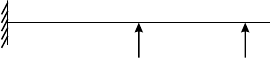4 M

2 (a) Determine the rotation and deflection at the free end of a cantilever beam shown in Fig Q2(a), by moment area method. Take EI-constant.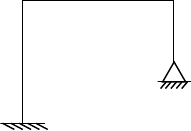10 M
2 (b) Determine the maximum slope and deflection for the given simply supported beams as shown in Fig. Q2(b), by conjugate beam method.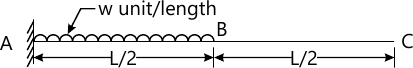10 M

3 (a) State Clark Maxwell's law of reciprocal deflection.
2 M
3 (b) Determine the vertical deflection at 'C' for the beam shown in Fig Q3 (b), by Castigliano's method.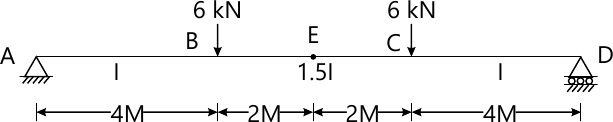9 M
3 (c) Determine the rotation at the free end of cantilever beam shown in Fig. Q3(c), by Castigliano's method.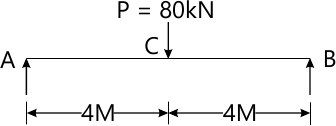9 M

4 (a) Find the vertical deflection at 'C' for the beam shown in Fig Q4 (a), by strain energy method.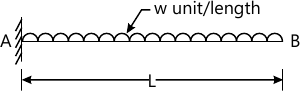8 M
4 (b) Determine the vertical deflection at 'C' for the frame loaded as shown in Fig. Q4 (b), by using unit load method. Cross sectional area of horizontal, vertical and inclined members are 1500, 2000 and 4000 mm2 respectively. Take EI=2.1×105 N/mm2.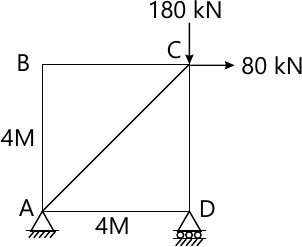12 M

5 A three hinged parabolic arch of span 24m, rise 6m with hinges at abutments and at crown point. Arch is subjected to a point load of 50 kN and 150kN at a distance of 8m and 20m from left support. Determine:
i) Reaction at supports.
ii) Resultant reaction and its inclination at supports.
iii) Bending moments at load and draw BMD.
iv) Normal thrust and radial shear at a distance of 6m from left and right supports.
20 M

6 A beam AB of span 4m is fixed at A and B carries a point load of 5kN at a distance of 1 m from end A. Calculate the support reactions by the method of consistent deformation. Also draw BMD and SFD. Take EI=constant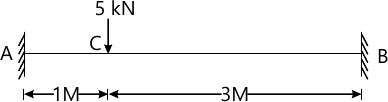20 M

7 Analyze the continuous beam by three moment equation. Find reactions and draw BMD and SFD.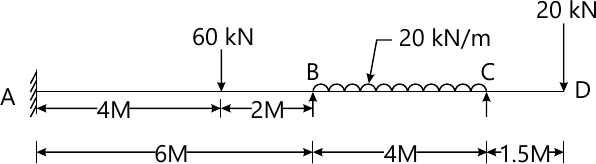20 M

8 A parabolic two hinged arch has span of 32m and a rise of 8m. A uniformly distributed load of 1 kN/m covers 8m horizontal length of the left side of the arch. If I=ID secθ, where θ is the inclination of the arch of the section to the horizontal, and I0 is the moment of inertia of the section at the crown. Find out the horizontal thrust at hinges and bending moment at 8m from the let hinge, Also, find the out normal thrust and radial shear at this section.
20 M

More question papers from Structural Analysis 1